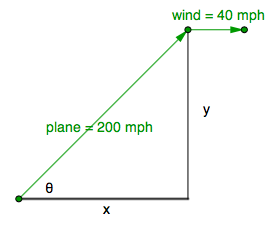### Home > PC > Chapter 10 > Lesson 10.2.3 > Problem10-103

10-103.

Write the appropriate parametric equations to find the position of a plane $t$ hours after takeoff if the plane is headed directly northeast at $200$ mph, and a wind is blowing east at $40$ mph.

Think vectors. What would the components be?

Draw and label a diagram.$\cos(\theta)=\frac{x}{200}$

Therefore $x = \:?$

The vectors in the diagram are velocity vectors.The problem asks for position. How do you calculate position given velocity?